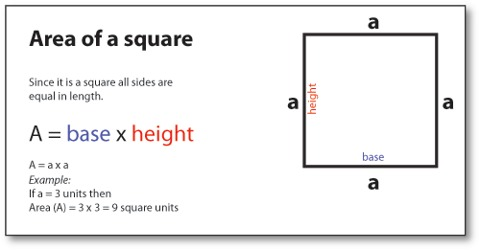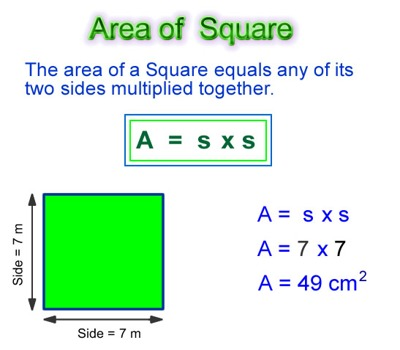Mathematic

# How to find the Area of a Square?Calculating the Area of a Square

Area is the size of a surface. A square has four sides all of equal length, and four corners, all right angles (90 degree angles). The number of square units required to fill the square is called the area of square.

The area of a square can be found by multiplying the base times itself. This is similar to the area of a rectangle but the base is the same length as the height. That means the number of square units it takes to completely fill a square. Formula: Width × Height.But since the width and height are by definition the same, the formula is usually written as

Area = s2

where s is the length of one side.

If a square has a base of length 6 cm its area is 7 × 7 = 49 square cm².